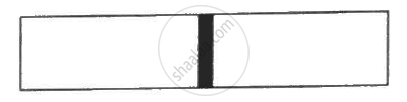Advertisement Remove all ads

# Figure Shows a Cylindrical Tube with Adiabatic Walls and Fitted with a Diathermic Separator. the Separator Can Be Slid in the Tube by an External Mechanism. an Ideal Gas is Injected - Physics

Sum

Figure shows a cylindrical tube with adiabatic walls and fitted with a diathermic separator. The separator can be slid in the tube by an external mechanism. An ideal gas is injected into the two sides at equal pressures and equal temperatures. The separator remains in equilibrium at the middle. It is now slid to a position where it divides the tube in the ratio of 1:3. Find the ratio of the pressures in the two parts of the vessel.

Use R=8.314J K-1 mol-1Advertisement Remove all ads

#### Solution

Since the separator initially divides the cylinder equally, the number of moles of gas are equal in the two parts. Thus,
n= n2= n

​Volume of the first part = V
Volume of the second part =​3V

It is given that the walls are diathermic. So, temperature of the two parts is equal. Thus,
T1 = T2 = T
Let pressure of first and second parts be P1 and P2, respectively.

For first part:- Applying equation of state, we get

$P_1 V = nRT ..........\left( 1 \right)$

For second part:- Applying equation of state, we get

$P_2 \left( 3V \right) = nRT .............\left( 2 \right)$

Dividing  eq. (1) by eq. (2), we get

$\frac{P_1 V}{P_2 \left( 3V \right)} = 1$

$\Rightarrow \frac{P_1}{P_2} = \frac{3}{1}$

$\Rightarrow P_1 : P_2 = 3: 1$

Concept: Kinetic Theory of Gases - Concept of Pressure
Is there an error in this question or solution?
Advertisement Remove all ads

#### APPEARS IN

HC Verma Class 11, Class 12 Concepts of Physics Vol. 2
Chapter 2 Kinetic Theory of Gases
Q 11 | Page 34
Advertisement Remove all ads
Advertisement Remove all ads
Share
Notifications

View all notifications

Forgot password?